## Example Questions

← Previous 1

### Example Question #2951 : Act Math

Which of the following is equivalent to cot(θ)sec(θ)sin(θ)

cot(θ)

tan(θ)

0

1

–sec(θ)

1

Explanation:

The first thing to do is to breakdown the meaning of each trig function, cot = cos/sin, sec = 1/cos, and sin = sin. Then put these back into the function and simplify if possible, so then (cos (Θ)/sin (Θ))*(1/cos (Θ))*(sin (Θ)) = (cos (Θ)*sin(Θ))/(sin (Θ)*cos(Θ)) = 1, since they all cancel out.

### Example Question #1 : How To Find A Reference Angle

Using trigonometry identities, simplify sinθcos2θ – sinθ

cos2θsinθ

cos3θ

None of these answers are correct

–sin3θ

sin2θcosθ

–sin3θ

Explanation:

Factor the expression to get sinθ(cos2θ – 1).

The trig identity cos2θ + sin2θ = 1 can be reworked to becomes cos2θ – 1 = –sinθ resulting in the simplification of –sin3θ.

### Example Question #1 : How To Find A Reference Angle

Using trig identities, simplify sinθ + cotθcosθ

tanθ

sin2θ

cscθ

cos2θ

secθ

cscθ

Explanation:

Cotθ can be written as cosθ/sinθ, which results in sinθ + cos2θ/sinθ.

Combining to get a single fraction results in (sin2θ + cos2θ)/sinθ.

Knowing that sin2θ + cos2θ = 1, we get 1/sinθ, which can be written as cscθ.

### Example Question #2952 : Act Math

Simplify sec4Θ – tan4Θ.

sec2Θ + tan2Θ

cosΘ – sinΘ

secΘ + sinΘ

tan2Θ – sin2Θ

sinΘ + cosΘ

sec2Θ + tan2Θ

Explanation:

Factor using the difference of two squares:  a2 – b2 = (a + b)(a – b)

The identity 1 + tan2Θ = sec2Θ which can be rewritten as 1 = sec2Θ – tan2Θ

So sec4Θ – tan4Θ = (sec2Θ + tan2Θ)(sec2Θ – tan2Θ) = (sec2Θ + tan2Θ)(1) = sec2Θ + tan2Θ

### Example Question #2 : How To Find A Reference Angle

Evaluate the expression below.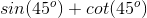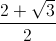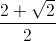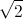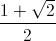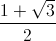Explanation:

At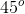, sine and cosine have the same value.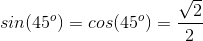Cotangent is given by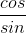.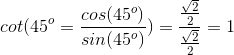Now we can evaluate the expression.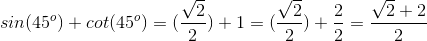### Example Question #3 : How To Find A Reference Angle

What is the reference angle of an angle that measures 3510 in standard  position?

109

90

369

351

90

Explanation:

3600 – 3510 = 90

### Example Question #1 : Reference Angles

Simplify the following expression: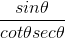cos2Θ

cscΘ

tanΘ

sin2Θ

None of the answers are correct

sin2Θ

Explanation:

Convert cotΘ and secΘ to sinΘ and cosΘ and simplify the resulting complex fraction.

cotΘ =    cosΘ             secΘ = 1

sinΘ               cosΘ

### Example Question #2 : How To Find A Reference Angle

What is the reference angle for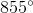?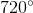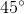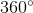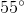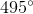Explanation:

The reference angle is between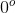and, starting on the positive-axis and rotating in a counter-clockwise manor.

To find the reference angle, we subtract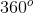for each complete revolution until we get a positive number less than.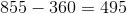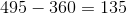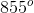is equal to two complete revolutions, plus a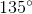angle. Sinceis in Quadrant II, we subtract it fromto get our reference angle: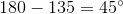### Example Question #3 : How To Find A Reference Angle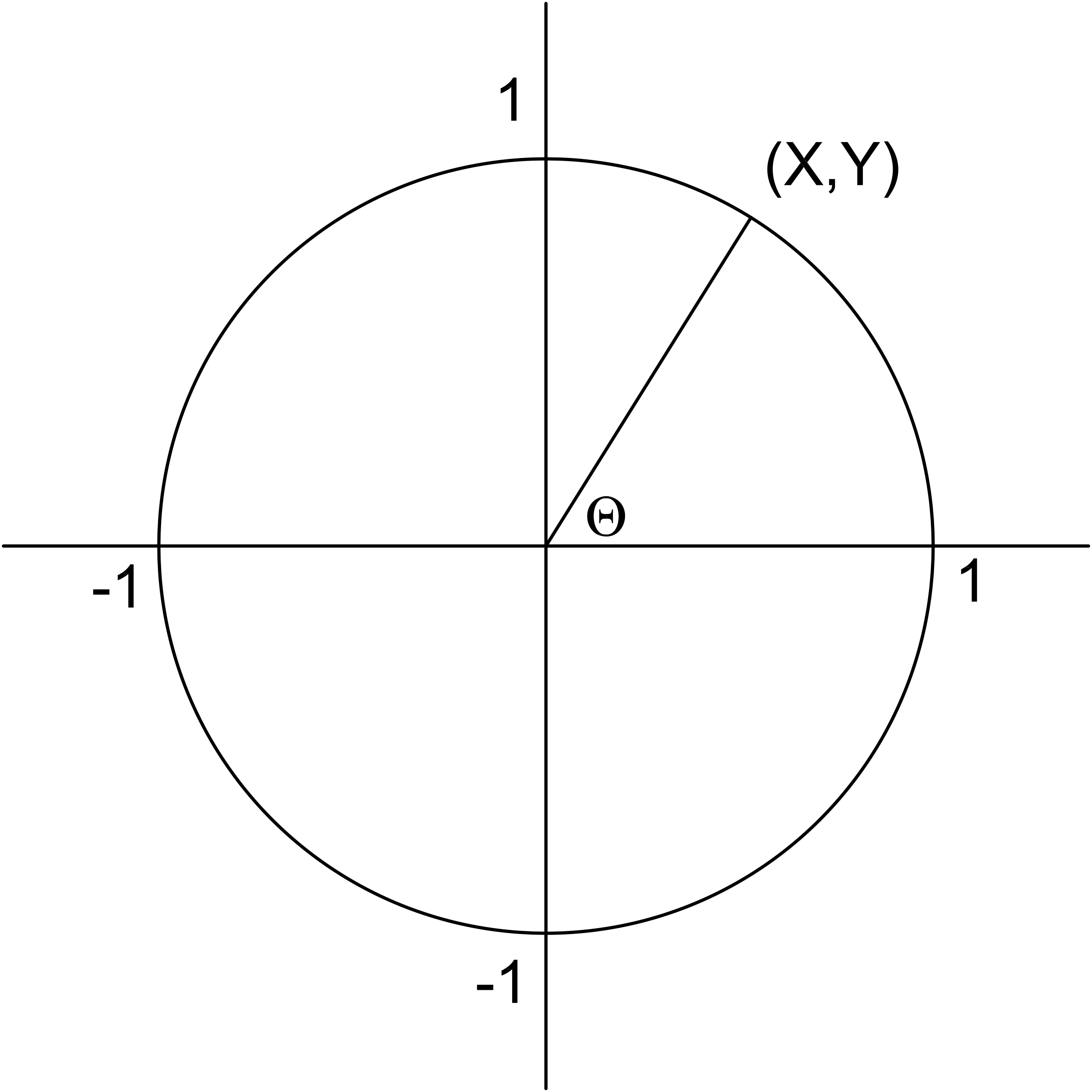In the unit circle above, if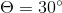, what are the coordinates of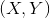?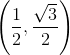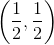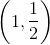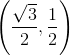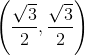Explanation:

On the unit circle, (X,Y) = (cos Θ, sin Θ).

(cos Θ,sin Θ) = (cos 30º, sin 30º) = (√3 / 2 , 1 / 2)

### Example Question #4 : How To Find A Reference Angle

What is the reference angle for?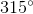Explanation:

A reference angle is the smallest possible angle between a given angle measurement and the x-axis.

In this case, our anglelies in Quadrant I, so the angle is its own reference angle.

Thus, the reference angle foris.

← Previous 1

### All ACT Math Resources